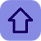# Frequently Asked Questions In C

Posted by Tushar BedekarQ1. Write a simple program to find the size of different basic data types in c?

Q.2 Write a program in c for showing working of different logical operator in C.

Q.3 Write a function to find the area of a triangle whose length of three sides is given.Q.4 Write a C program to read the internal test marks of 25 students in a class and show the number of students who have scored more than 50% in the test.

Q.5 Write a C function to swap two given numbers using call by reference mechanism?

Q.6 Write a C program for finding GCD of two given numbers.

Q.7 Write a C program for addition of two 3x3 matrix.

Q.8 Write a C programme for counting the number of words in a given string?

Q.9 Write a C programme for concatenating two given strings?

Q.10 Write a C program using structure to find students grades in a class?

Q.11 Write a program to insert any 10 numbers and calculate its EVEN SUM,ODD SUM and EVEN AVERAGE,ODD AVERAGE.

Q.12 Write a program to insert any ten numbers and find minimum and maximum numbers.

Q.13 Write a program to calculate a given number is a prime number or not.

Q.14 Write a program to swapping two values using pointer and malloc function.

Q.15 C program for malloc function with example.